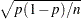Displayed Output for Exact Analysis

If an exact analysis is requested with an EXACT statement, the displayed output includes the following tables. If the METHOD=NETWORKMC option is specified, the test and estimate tables are renamed "Monte Carlo" tables and a Monte Carlo standard error column () is displayed.

### Sufficient Statistics

Displays if you request an OUTDIST= data set in an EXACT statement. The table lists the parameters and their observed sufficient statistics.

### (Monte Carlo) Conditional Exact Tests

This table tests the hypotheses that the parameters of interest are insignificant. See the section Exact Logistic and Exact Poisson Regression for details.

### (Monte Carlo) Exact Parameter Estimates

Displays if you specify the ESTIMATE option in the EXACT statement. This table gives individual parameter estimates for each variable (conditional on the values of all the other parameters in the model), confidence limits, and a two-sided p-value (twice the one-sided p-value) for testing that the parameter is zero. See the section Exact Logistic and Exact Poisson Regression for details.

### (Monte Carlo) Exact Odds Ratios

Displays if you specify the ESTIMATE=ODDS or ESTIMATE=BOTH option in the EXACT statement. See the section Exact Logistic and Exact Poisson Regression for details.

### Strata Summary

Displays if a STRATA statement is also specified. Shows the pattern of the number of events and the number of nonevents, or of the number of observations, in a stratum. See the section STRATA Statement for more information.

### Strata Information

Displays if a STRATA statement is specified with the INFO option.